Hits: 3

# Decrease Key and Delete Node Operations on a Fibonacci Heap

#### In this tutorial, you will learn how decrease key and delete node operations work. Also, you will find working examples of these operations on a fibonacci heap in Python.

In a fibonacci heap, decrease-key and delete-node are important operations. These operations are discussed below.

## Decreasing a Key

In decreasing a key operation, the value of a key is decreased to a lower value.

Following functions are used for decreasing the key.

### Decrease-Key

1. Select the node to be decreased, x, and change its value to the new value k.
2. If the parent of xy, is not null and the key of parent is greater than that of the k then call `C``ut(x)` and `C``ascading-``C``ut(y)` subsequently.
3. If the key of x is smaller than the key of min, then mark x as min.

### Cut

1. Remove x from the current position and add it to the root list.
2. If x is marked, then mark it as false.

### Cascading-Cut

1. If the parent of y is not null then follow the following steps.
2. If y is unmarked, then mark y.
3. Else, call `Cut(y)` and `Cascading-Cut(parent of y)`.

## Decrease Key Example

The above operations can be understood in the examples below.

### Example: Decreasing 46 to 15.

1. Decrease the value 46 to 15.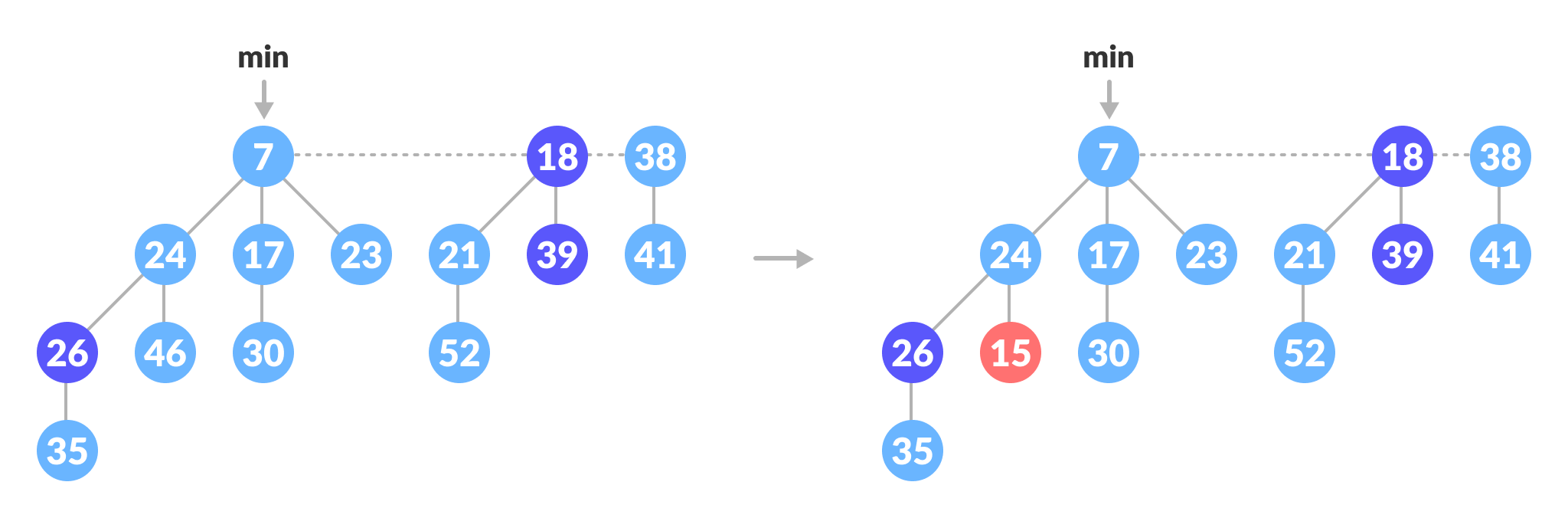Decrease 46 to 15
2. Cut part: Since `24 ≠ nill` and `15 < its parent`, cut it and add it to the root list. Cascading-Cut part: mark 24.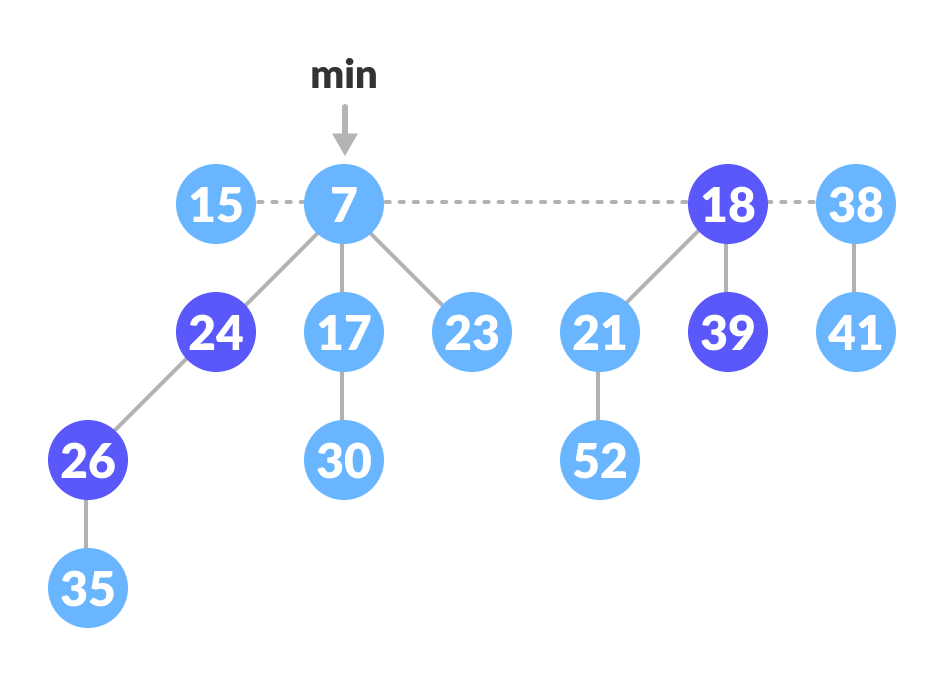Add 15 to root list and mark 24

### Example: Decreasing 35 to 5

1. Decrease the value 35 to 5.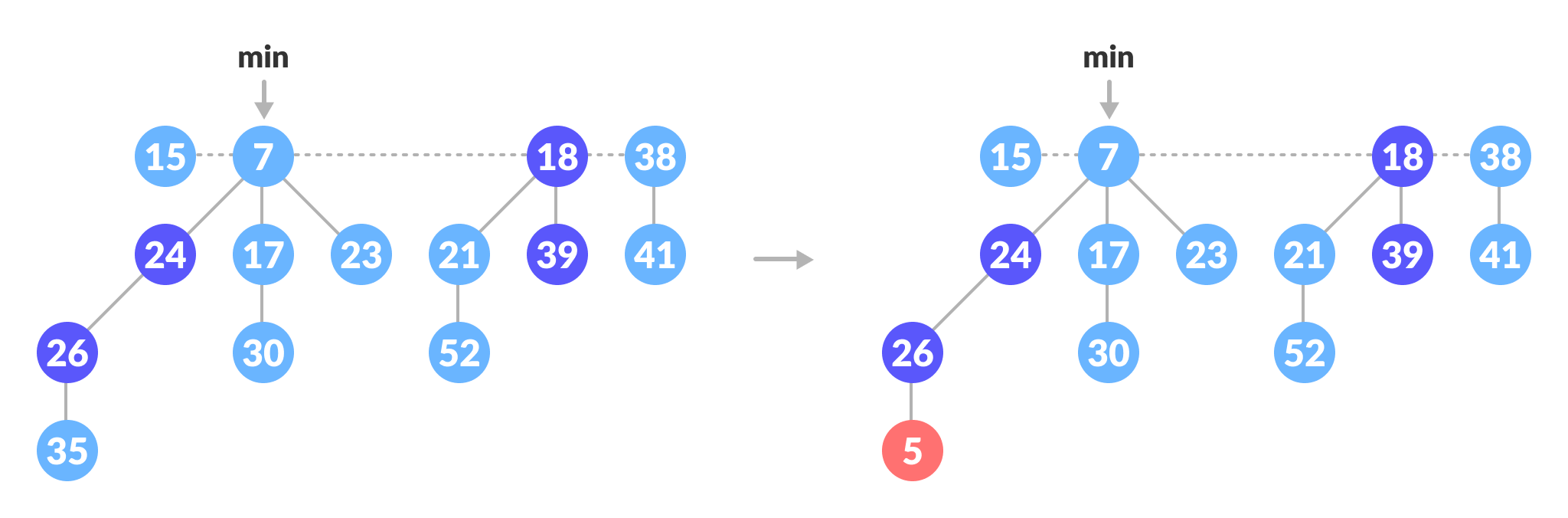Decrease 35 to 5
2. Cut part: Since `26 ≠ nill` and `5<its parent`, cut it and add it to the root list.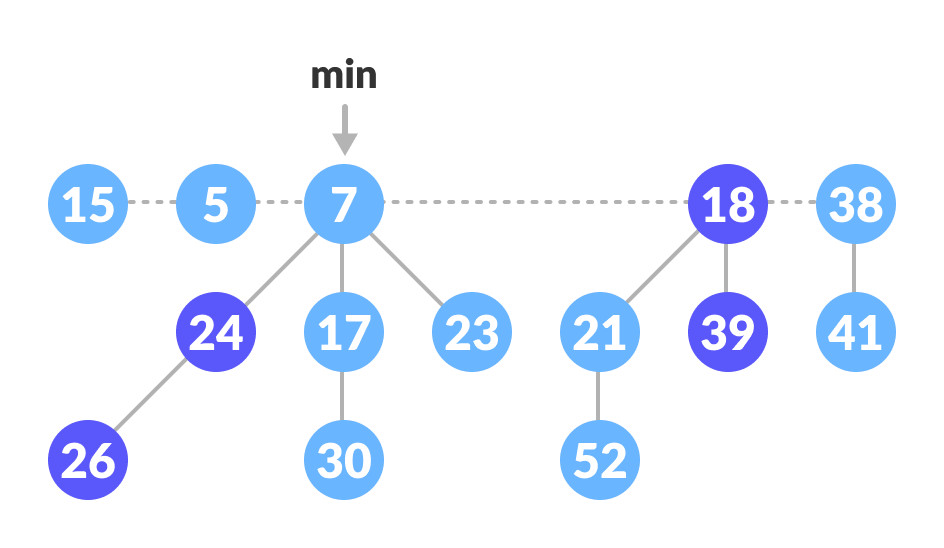Cut 5 and add it to root list
3. Cascading-Cut part: Since 26 is marked, the flow goes to `Cut` and `Cascading-Cut`.
Cut(26): Cut 26 and add it to the root list and mark it as false.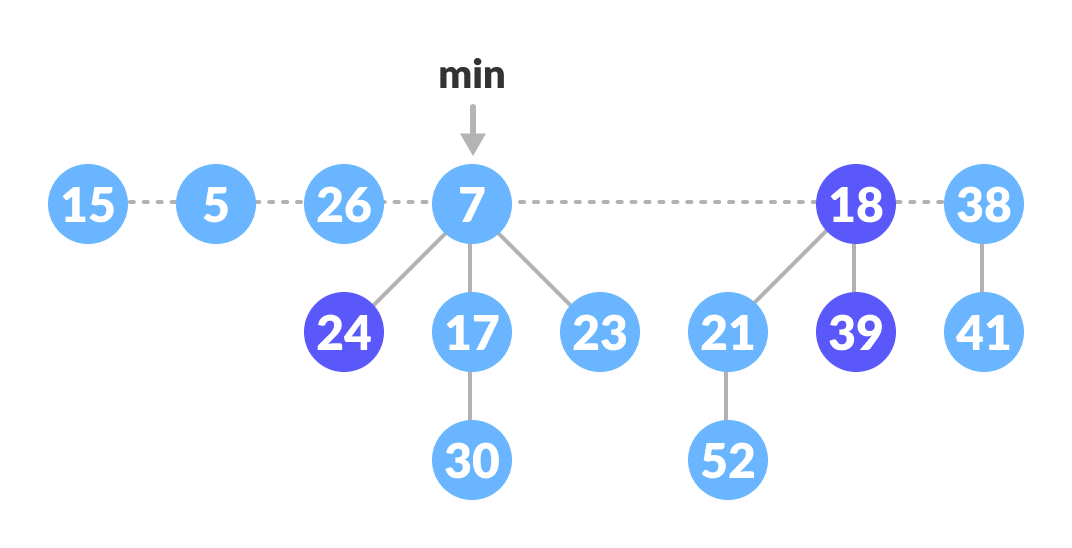Cut 26 and add it to root list

Cascading-Cut(24):
Since the 24 is also marked, again call `Cut(24)` and `Cascading-Cut(7)`. These operations result in the tree below.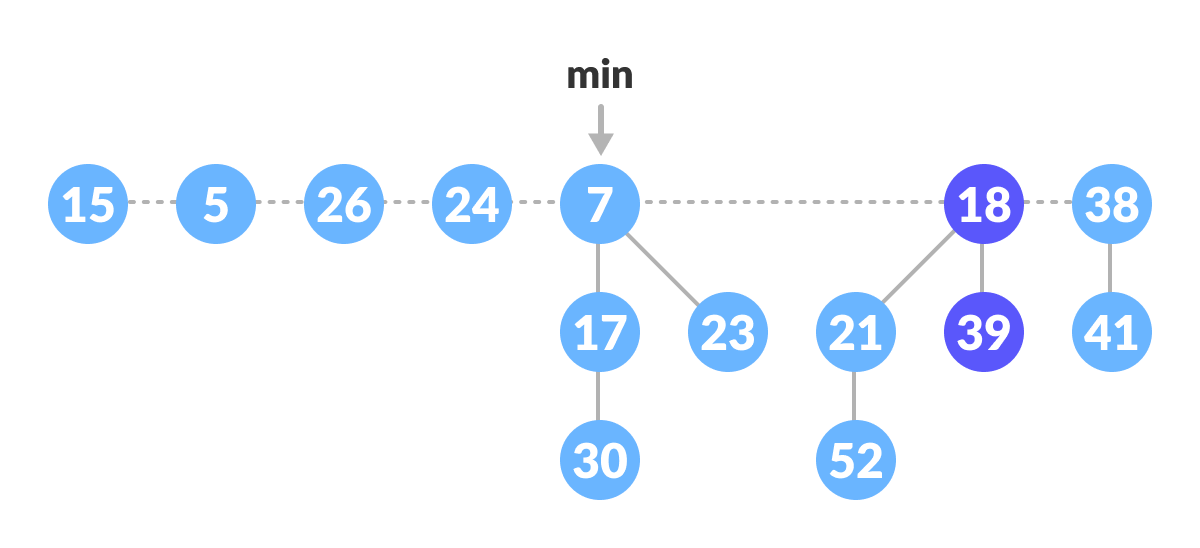Cut 24 and add it to root list
4. Since `5 < 7`, mark 5 as min.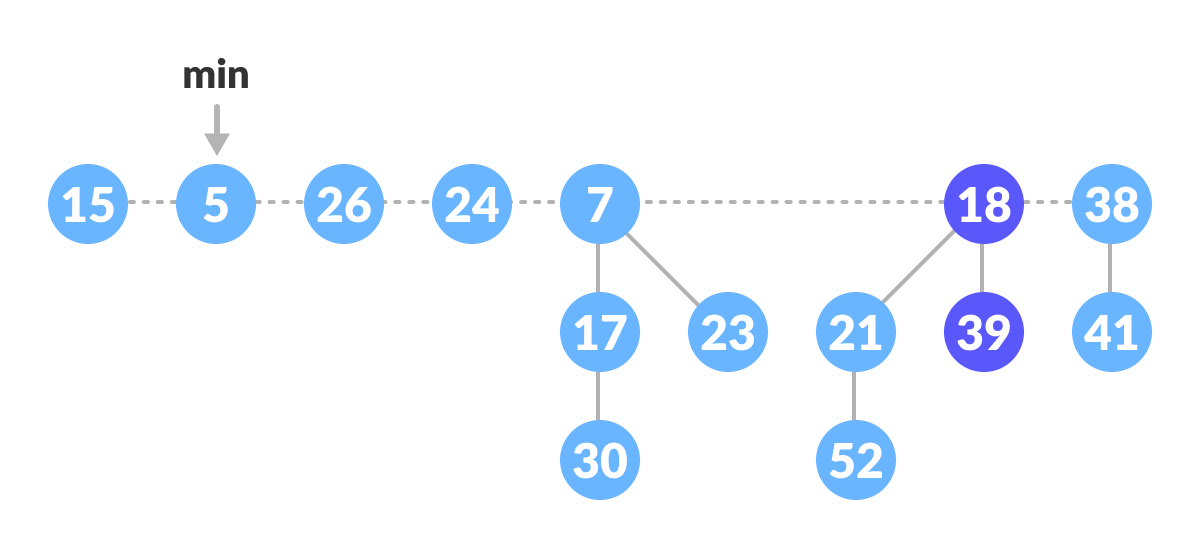Mark 5 as min

## Deleting a Node

This process makes use of decrease-key and extract-min operations. The following steps are followed for deleting a node.

1. Let k be the node to be deleted.
2. Apply decrease-key operation to decrease the value of k to the lowest possible value (i.e. -∞).
3. Apply extract-min operation to remove this node.

## Python Examples

``````/* Fibonacci Heap in python */

import math

class FibonacciTree:
def __init__(self, key):
self.key = key
self.children = []
self.order = 0

def add_at_end(self, t):
self.children.append(t)
self.order = self.order + 1

class FibonacciHeap:
def __init__(self):
self.trees = []
self.least = None
self.count = 0

def insert(self, key):
new_tree = FibonacciTree(key)
self.trees.append(new_tree)
if (self.least is None or key < self.least.key):
self.least = new_tree
self.count = self.count + 1

def get_min(self):
if self.least is None:
return None
return self.least.key

def extract_min(self):
smallest = self.least
if smallest is not None:
for child in smallest.children:
self.trees.append(child)
self.trees.remove(smallest)
if self.trees == []:
self.least = None
else:
self.least = self.trees
self.consolidate()
self.count = self.count - 1
return smallest.key

def consolidate(self):
aux = (floor_log2(self.count) + 1) * [None]

while self.trees != []:
x = self.trees
order = x.order
self.trees.remove(x)
while aux[order] is not None:
y = aux[order]
if x.key > y.key:
x, y = y, x
x.add_at_end(y)
aux[order] = None
order = order + 1
aux[order] = x

self.least = None
for k in aux:
if k is not None:
self.trees.append(k)
if (self.least is None
or k.key < self.least.key):
self.least = k

def floor_log2(x):
return math.frexp(x) - 1

fheap = FibonacciHeap()

fheap.insert(11)
fheap.insert(10)
fheap.insert(39)
fheap.insert(26)
fheap.insert(24)

print('Minimum value: {}'.format(fheap.get_min()))

print('Minimum value removed: {}'.format(fheap.extract_min()))``````

## Complexities

 Decrease Key O(1) Delete Node O(log n)

# Special 95% discount

## 2000+ Applied Machine Learning & Data Science Recipes

### Portfolio Projects for Aspiring Data Scientists: Tabular Text & Image Data Analytics as well as Time Series Forecasting in Python & R## Two Machine Learning Fields

There are two sides to machine learning:

• Practical Machine Learning:This is about querying databases, cleaning data, writing scripts to transform data and gluing algorithm and libraries together and writing custom code to squeeze reliable answers from data to satisfy difficult and ill defined questions. It’s the mess of reality.
• Theoretical Machine Learning: This is about math and abstraction and idealized scenarios and limits and beauty and informing what is possible. It is a whole lot neater and cleaner and removed from the mess of reality.

Data Science Resources: Data Science Recipes and Applied Machine Learning Recipes

Introduction to Applied Machine Learning & Data Science for Beginners, Business Analysts, Students, Researchers and Freelancers with Python & R Codes @ Western Australian Center for Applied Machine Learning & Data Science (WACAMLDS) !!!

Latest end-to-end Learn by Coding Recipes in Project-Based Learning:

Applied Statistics with R for Beginners and Business Professionals

Data Science and Machine Learning Projects in Python: Tabular Data Analytics

Data Science and Machine Learning Projects in R: Tabular Data Analytics

Python Machine Learning & Data Science Recipes: Learn by Coding

R Machine Learning & Data Science Recipes: Learn by Coding

Comparing Different Machine Learning Algorithms in Python for Classification (FREE)

`Disclaimer: The information and code presented within this recipe/tutorial is only for educational and coaching purposes for beginners and developers. Anyone can practice and apply the recipe/tutorial presented here, but the reader is taking full responsibility for his/her actions. The author (content curator) of this recipe (code / program) has made every effort to ensure the accuracy of the information was correct at time of publication. The author (content curator) does not assume and hereby disclaims any liability to any party for any loss, damage, or disruption caused by errors or omissions, whether such errors or omissions result from accident, negligence, or any other cause. The information presented here could also be found in public knowledge domains.  `